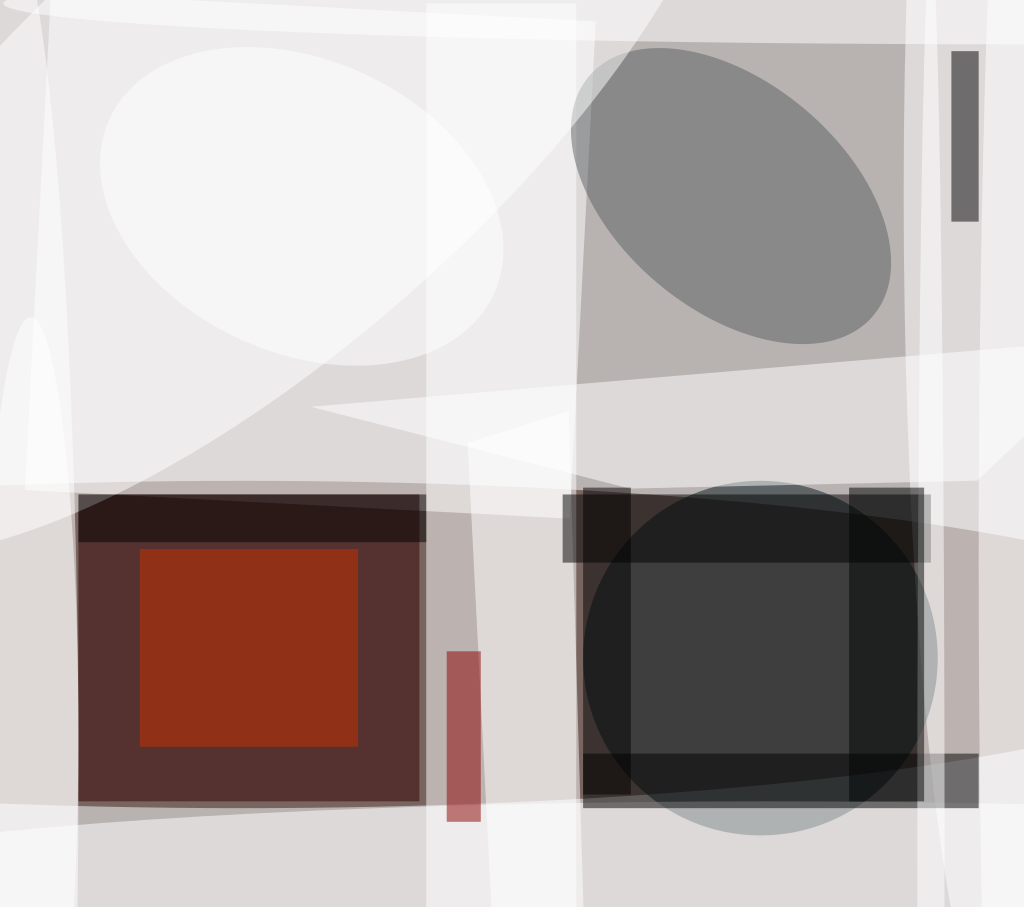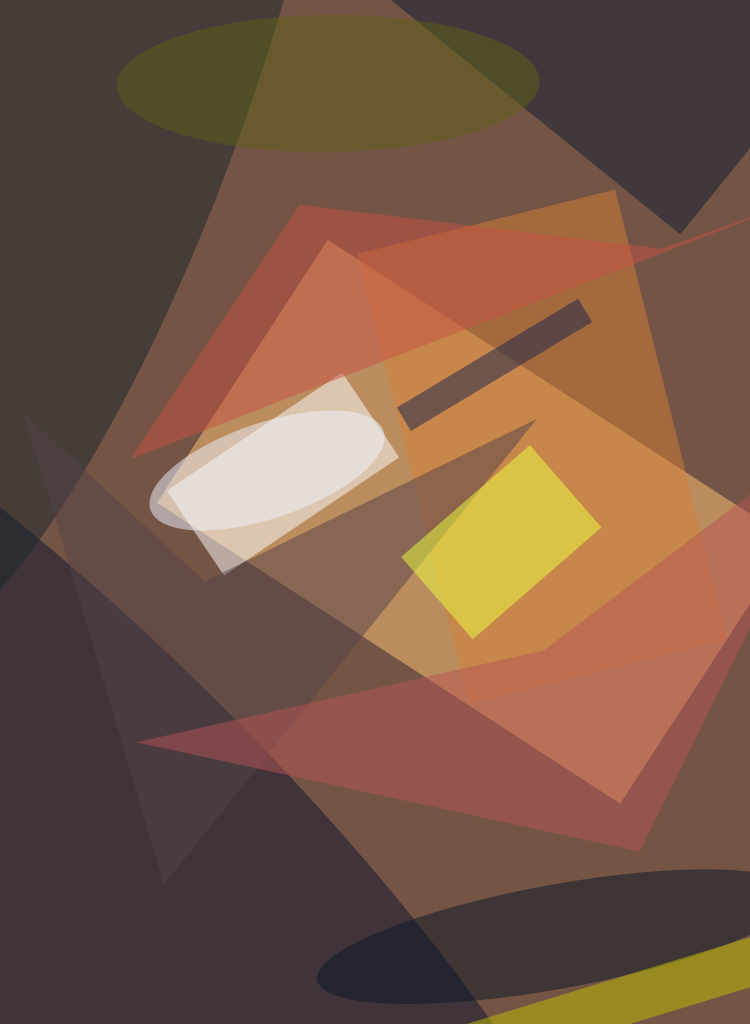Random reflectors of a proposed type would be fabricated on the back surfaces of quantum-well infrared photodetectors (QWIPs) to increase the coupling efficiency of infrared light into the QWIPs in two wavelength bands. A reflective surface according to this proposal would comprise an array of square cells, each cell divided into a 2-by-2 array of subcells that would lie at various, partly random depths relative to the nominal flat back surface of the QWIP. The subcells at the various depths would be formed by one of several lithographic processes, depending on the specific design. In comparison with the light-scattering efficiency of crossed-diffraction-grating QWIP light couplers that have been reported previously in NASA Tech Briefs, the light-scattering efficiency of the proposed random reflectors would be less dependent on wavelength; this would be an advantage in QWIP applications in which there are requirements for broad-band or multiband response.

As discussed in more detail in several prior articles in NASA Tech Briefs, in order to couple light into a QWIP, the quantum-well layers of which lie parallel to its focal plane, it is necessary to cause light to propagate within the QWIP along a direction other than perpendicular to the focal plane; the efficiency of coupling increases with the angle between the direction of propagation and the perpendicular. Most of the incoming light is concentrated within a small angular range about the perpendicular. Therefore, the purpose served by the proposed random reflectors and by the previously reported cross-grating QWIP light couplers is to exploit diffraction to divert the incoming light, as efficiently as possible, to propagation at large angles from the perpendicular. Additionally, light that is not absorbed will get reflected and encounter a different structure on each bounce. This improves the ability of the QWIP to trap the light and absorb a larger fraction of photons.The Zero-Angle Reflectivity Spectrum and the Diffraction Pattern were computed for the reflector pattern shown at the top. (The actual relfectivity would likely be greater but similar in shape.) The computations were based on free-space wavelengths of 14 and 16 µm, a subcell (reflector-grid) interval of 2.5 µm, and an index of refraction of QWIP material of 3.1. A free-space wavelength of 15 µm was used in calculating the diffraction pattern.

For maximum diffraction at high angles, the subcells would be given dimensions of the order of the wavelengths of interest. It would also be necessary to minimize low-angle diffraction in order to minimize waste of light. Therefore, the depths of the subcells would be chosen so that at the middle wavelengths of both wavelength bands of interest, any light propagating along the perpendicular direction would interfere destructively. Specifically, the depths of the four subcells (di, i = 1 to 4) of a given cell would be as follows:

d1 = a random value between 0 and λ1/4,

d2 = d1 + λ1/4,

d3 = d1 + λ2/4, and

d4 = d1 + λ1/4 + λ2/4,

where λ1 and λ2 are the two wavelengths of interest inside the QWIP material.

This choice of depths would cause the subcells to act in pairs to produce destructive interference: At λ1, destructive interference would occur between subcells 1 and 2 and between subcells 3 and 4; at λ2, destructive interference would occur between subcells 1 and 3 and between subcells 2 and 4.

The figure shows a gray-scale representation of a reflector pattern of this type, a plot of the computed zero-angle (perpendicular) reflectivity, and a gray-scale representation of the computed diffraction magnitude as a function of the sine of the angular deviation (θ) from the perpendicular. All waves inside the circle of radius sin(θ) = 1 are propagating, and those outside the circle are evanescent. As needed for efficient coupling, the computed zero-angle reflectivity goes to zero at the two design wavelengths and the computed diffraction magnitude at high angles is strong.

This work was done by Daniel Wilson, Sumith Bandara, Sarath Gunapala, and John K. Liu of Caltech for NASA's Jet Propulsion Laboratory.

In accordance with Public Law 96-517, the contractor has elected to retain title to this invention. Inquiries concerning rights for its commercial use should be addressed to

###### Technology Reporting OfficeJPLMail Stop 122-1164800 Oak Grove DrivePasadena, CA 91109(818) 354-2240

Refer to NPO-20682##### Photonics Tech Briefs Magazine

This article first appeared in the May, 2000 issue of Photonics Tech Briefs Magazine.

Read more articles from the archives here.